#### How to stop Excel from doing numbers E+?

In this lesson, you will learn how to disable scientific notation (e.g., 1,23457E+17) in an Excel spreadsheet.

This problem exists when you want to paste a long number. Pasting it to the spreadsheet, Excel changes the formatting to scientific notation (e.g. 1,23457E+17).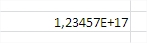This problem is caused by Excel, which has a 15-digit precision limit. It’s annoying, because most of the time, I’d prefer it if Excel just treated the number as text (until I wanted it sorted). You can solve this problem.

## How to format the cell to disable scientific notation?

Just right click on the cell and choose Format cell.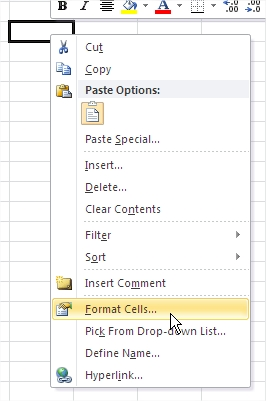Change the format from General to Number with a zero number of decimal places.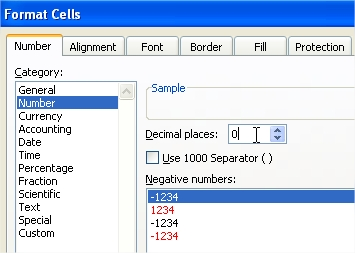Now, this problem doesn’t exist any more.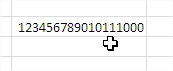Tips:

• This solution works for other types of formatting as well. For example, Custom with a single 0 or Text.
• The number format does not affect the actual cell value, which is displayed in the formula bar.
• When Excel displays a cell with #### signs, it means that the cell is too narrow. Just make it wider.

## Increasing the number of decimal places

Increasing the number of decimal places is one of the simplest and most straightforward ways to disable scientific notation in Excel.

1. In the Format Cells dialog box, select the “Number” tab.
2. In the “Number” section, select the number of decimal places you want to display. The default is 2 decimal places, but you can increase this number to show more decimal places if necessary.
3. The number will be displayed in the specified number of decimal places, instead of in scientific notation.

Note: This method works best for numbers that are small enough to be displayed with the specified number of decimal places. If the number is too large, it may still be displayed in scientific notation even if you increase the number of decimal places. In such cases, you may need to use a different method, such as changing the cell format to “Text” or using the “Text” formula.

## Using the Custom format

Using the “Custom” format is another way to disable scientific notation in Excel. This method involves specifying a custom format for the cell to display the number in a specific way.

1. In the Format Cells dialog box, select the “Number” tab.
2. In the “Category” section, select “Custom.”
3. In the “Type” field, enter a custom format for the number. For example, to display the number with no decimal places, enter “0”. To display the number with 2 decimal places, enter “0.00”.
4. The number will be displayed in the specified format, instead of in scientific notation.

Note: This method can be useful when you need to display a specific number of decimal places or when you want to display the number in a specific way (such as with a comma separator).

## Using the ROUND formula

Using the “Round” formula is a way to disable scientific notation in Excel by rounding the number to a specified number of decimal places.

1. Enter the formula =ROUND(A1,0), where A1 is the cell that contains the number in scientific notation, and 0 is the number of decimal places you want to round to.
2. The number in cell A1 will be rounded to the specified number of decimal places and displayed in the selected cell.

Note: The “Round” formula is a useful method when you want to display the number in a simplified form with a specified number of decimal places. However, be aware that rounding can result in the loss of precision, and it may not be suitable for all purposes.

## How to concatenate the cell to disable scientific notation?

The other way is to concatenate the cells to display the full content of each cell.

A simple =CONCATENATE(A1) formula would do the trick. Thanks to that trick, you will not see “E+” any more.

It worked because that is how the concatenate function works. As an output, it creates a single string, and Excel does not apply scientific notation to such a string. It is worth mentioning that it does not change the format of the cell.

## Using the Text to Columns Tool

You can also remove scientific notation with the Text to Columns tool. This tool is used to improve the content of cells in case of problems with their content or formatting.

To start, select the column that contains unnecessary scientific notations and go to the Excel ribbon. Click Data > Text to Columns.

In the first step, check the Fixed width option. Already at this stage you can see in the preview of selected data at the bottom of the window that scientific notation will be deleted.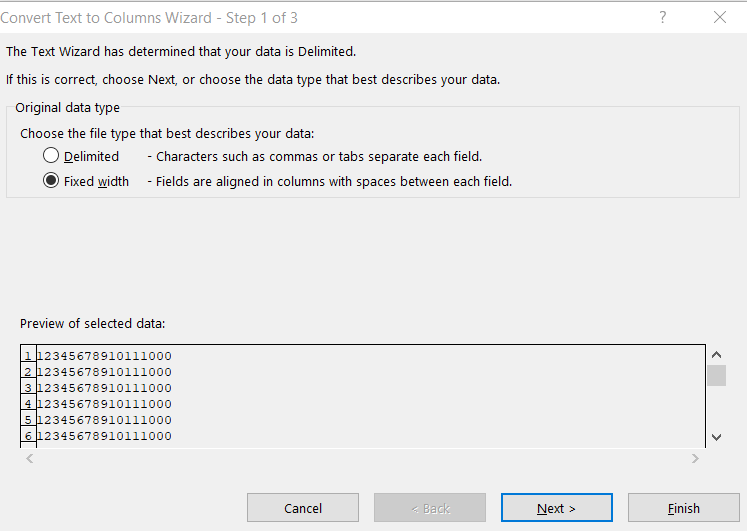In the third step, select the text from available Excel data types. The scientific notation will disappear from the cells.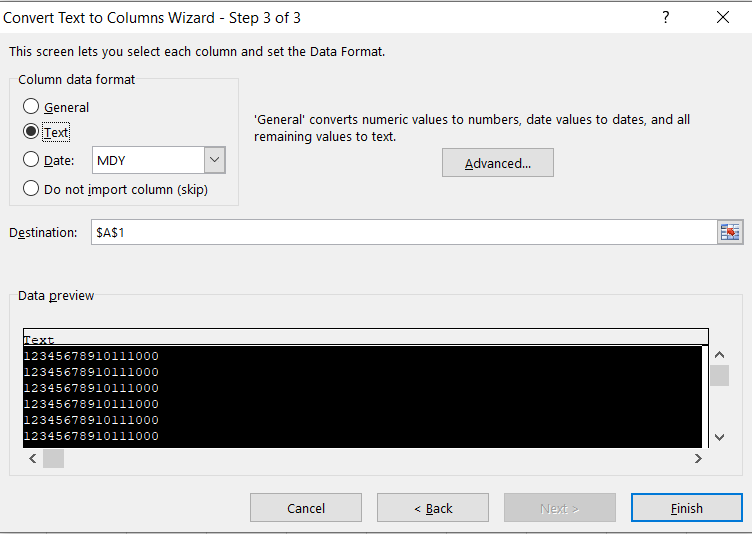The inconvenience is that green triangles will appear in the cells. If they bother you, check this link on how to remove green triangles from a spreadsheet in Excel.

## Conversion of the cell to disable scientific notation

There is one more tricky way to accomplish the expected output that I would like to share with you.

There is a way to convert the content of the cell to the specific number format without changing it. Excel would stop using the scientific notation as well.

=TEXT(A1,”0″) is the Excel formula you should use.

The result is the same. “E+” disappeared from the cell.

## Trimming to remove scientific notation

Another way to remove scientific notation from your workbook is to use the trim function.

The Trim function is used to remove excess spaces from a cell. Using the formula Trim only leaves single spaces between words.

As a side effect of using the Trim formula, you will get rid of scientific notation.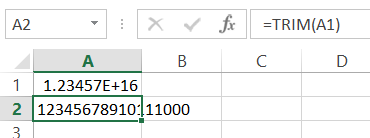Use the formula = TRIM (A1) to get rid of scientific notation.

## Using the IF function

Using the “IF” formula is a way to disable scientific notation in Excel by evaluating the number and displaying it in either scientific notation or a custom format.

1. Enter the formula =IF(ABS(A1)<1,”0.00″,A1), where A1 is the cell that contains the number in scientific notation.
2. The formula will evaluate the number in cell A1 and determine whether it is less than 1. If it is less than 1, it will display the number in a custom format with two decimal places. If it is not less than 1, it will display the number in its original format.

Note: This method is useful when you want to display the number in a custom format based on its value. However, be aware that the custom format may not be suitable for all purposes, and you may need to modify the formula to meet your specific needs.

## Using the FIX function

Using the “FIX” formula is a way to disable scientific notation in Excel by rounding the number down to a specified number of decimal places.

1. Enter the formula =FIX(A1,n), where A1 is the cell that contains the number in scientific notation and n is the number of decimal places you want to round down to.
2. The number in cell A1 will be rounded down to the specified number of decimal places, and scientific notation will be disabled.

Note: This method is useful when you want to round the number and display it in a more readable format.

## Using the INT function

Using the “INT” formula is a way to disable scientific notation in Excel by rounding the number down to the nearest integer.

1. Enter the formula =INT(A1), where A1 is the cell that contains the number in scientific notation.
2. The number in cell A1 will be rounded down to the nearest integer, and scientific notation will be disabled.

Note: This method is useful when you want to round the number and display it as a whole number.

## Using the TRUNC function

Using the “TRUNC” formula is a way to disable scientific notation in Excel by truncating the number to a specified number of decimal places.

1. Enter the formula =TRUNC(A1,n), where A1 is the cell that contains the number in scientific notation and n is the number of decimal places you want to keep.
2. The number in cell A1 will be truncated to the specified number of decimal places, and scientific notation will be disabled.

Note: This method is useful when you want to remove the decimal portion of the number and display it as a whole number or a number with a limited number of decimal places.

## Pasting values as text

Pasting values as text is a way to disable scientific notation in Excel by converting the number to text.

1. Right-click the selected cells and choose “Copy”.
2. Right-click the target cell where you want to paste the copied numbers and choose “Paste Special”.
3. Select “Values” and “Text” as the paste options.
4. The numbers in scientific notation will be converted to text and scientific notation will be disabled.

Note: This method is useful when you want to preserve the original format of the numbers and prevent them from being changed by Excel.